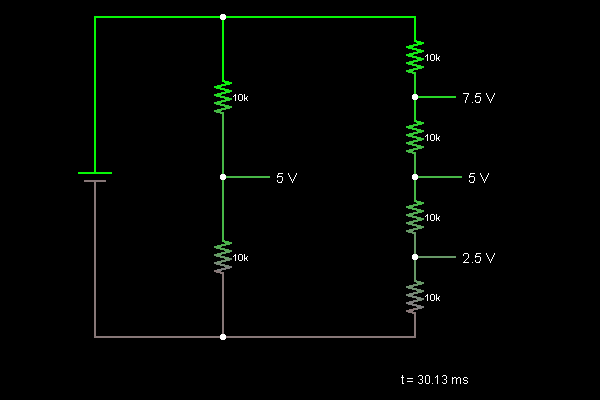# Circuit Simulator - Voltage Divider

Voltage DividerCircuit Description:
This is a voltage divider, a simple circuit that can be used to derive a reference voltage from a known supply voltage. In the middle, two equal resistors generate a 5 V voltage from the 10 V supply. On the right, four resistors provide 7.5 V, 5 V, and 2.5 V.

Discussion:
9 comments Page 1 of 1.

Vis lin said:   8 years ago
How to build a connection of 12 AC Fan(220~240V;0.09A)?
(1)

Million said:   10 years ago
I need brief explanation about mesh analysis of current and voltage.
(6)

How to generate +2.5 and -2.5 voltages from single +3 Volts Battery?
(7)

How to use of thevenin theorem in practical?
(3)

How many resistors should we use to divide 5 volts into 1 volts across each resistor?
Is it 5?
(7)

Give me clear information of the thevinin's and norton's theorem.
(6)

Hey. If two volatge sources connected in parallel then the current flow will be the same.
(1)